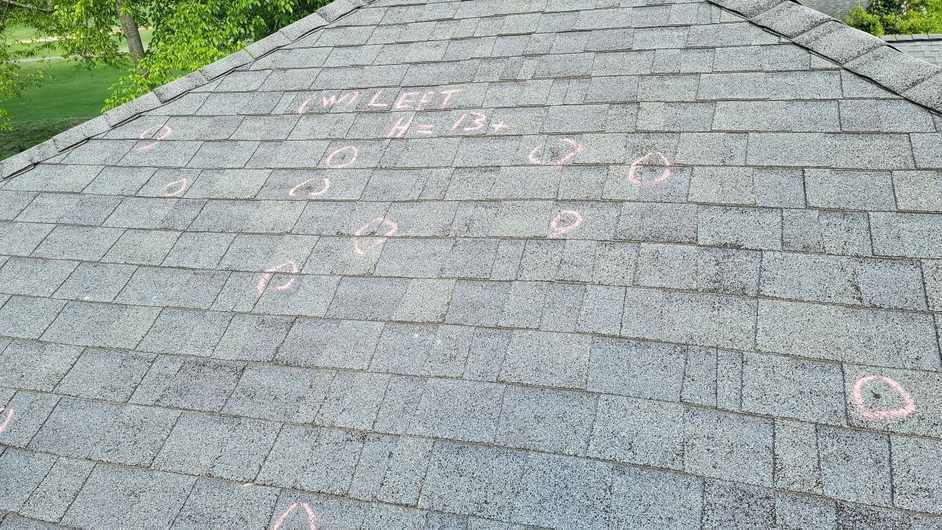## What is a Roofing Square?

A roofing square is a unit of measurement used in the roofing industry to determine the size of a roof.

It is equal to 100 square feet or a 10-foot by 10-foot area.

Roofing squares are commonly used to estimate the amount of materials needed for a roofing project.### How is a Roofing Square Calculated?

To calculate the number of roofing squares needed for a project, you need to measure the length and width of the roof in feet.

Multiply the length by the width to get the total square footage.

Then, divide the total square footage by 100 to determine the number of roofing squares required.

### What is the Average Size of a Roofing Square?

The average size of a roofing square is 100 square feet.

However, the actual size may vary depending on the specific requirements of the project.

Some roofs may be larger or smaller than the standard size, which can affect the number of roofing squares needed.

### How Big is a Roofing Square in 2023?

In 2023, the size of a roofing square remains the same as it has been for many years – 100 square feet.

There have been no significant changes in the industry standards regarding the size of a roofing square.

### Why is a Roofing Square Important?

A roofing square is important because it helps contractors and homeowners estimate the amount of materials needed for a roofing project.

By knowing the size of the roof in terms of squares, they can accurately calculate the quantity of shingles, underlayment, and other materials required, which can help prevent over or under-ordering.## Factors Affecting the Size of a Roofing Square

While the standard size of a roofing square is 100 square feet, there are several factors that can affect the actual size of a roof and, consequently, the number of roofing squares needed for a project.

These factors include:

• Roof pitch: The steepness or slope of a roof can impact its size. A roof with a higher pitch will have a larger surface area and may require more roofing squares.
• Roof shape: The shape of a roof, such as gable, hip, or flat, can also affect its size. Different roof shapes have different surface areas, which can influence the number of roofing squares needed.
• Roof features: The presence of chimneys, skylights, vents, and other roof features can alter the size of a roof. These features create additional surface areas that need to be accounted for when calculating the number of roofing squares required.
• Waste factor: A waste factor is often added to the total square footage to account for material waste during installation. This can increase the number of roofing squares needed.
• Local building codes: Local building codes may have specific requirements for roof sizes, which can impact the number of roofing squares needed for a project.## How to Calculate the Number of Roofing Squares Needed

To calculate the number of roofing squares needed for a project, follow these steps:

1. Measure the length and width of the roof in feet.
2. Multiply the length by the width to get the total square footage.
3. Divide the total square footage by 100 to determine the number of roofing squares required.
4. Consider any additional factors, such as roof pitch, shape, features, waste factor, and local building codes, that may affect the size and number of roofing squares needed.## Common Roofing Square Sizes

While the standard size of a roofing square is 100 square feet, there are common variations in roof sizes that can affect the number of roofing squares needed.

Some common roofing square sizes include:

• 10 feet by 10 feet (100 square feet)
• 20 feet by 5 feet (100 square feet)
• 25 feet by 4 feet (100 square feet)
• 50 feet by 2 feet (100 square feet)
• 100 feet by 1 foot (100 square feet)## Conclusion

A roofing square is a unit of measurement used in the roofing industry to determine the size of a roof.

The standard size of a roofing square is 100 square feet, and this has not changed in 2023.

However, factors such as roof pitch, shape, features, waste factor, and local building codes can affect the actual size of a roof and the number of roofing squares needed for a project.

By accurately calculating the number of roofing squares required, contractors and homeowners can ensure they order the right amount of materials for their roofing projects.